# Detect, Classify, and Track Vehicles Using Lidar

This example shows how to detect, classify, and track vehicles by using lidar point cloud data captured by a lidar sensor mounted on an ego vehicle. The lidar data used in this example is recorded from a highway-driving scenario. In this example, the point cloud data is segmented to determine the class of objects using the `PointSeg` network. A joint probabilistic data association (JPDA) tracker with an interactive multiple model filter is used to track the detected vehicles.

### Overview

The perception module plays an important role in achieving full autonomy for vehicles with an ADAS system. Lidar and camera are essential sensors in the perception workflow. Lidar is good at extracting accurate depth information of objects, while camera produces rich and detailed information of the environment which is useful for object classification.

This example mainly includes these parts:

• Ground plane segmentation

• Semantic segmentation

• Oriented bounding box fitting

• Tracking oriented bounding boxes

The flowchart gives an overview of the whole system.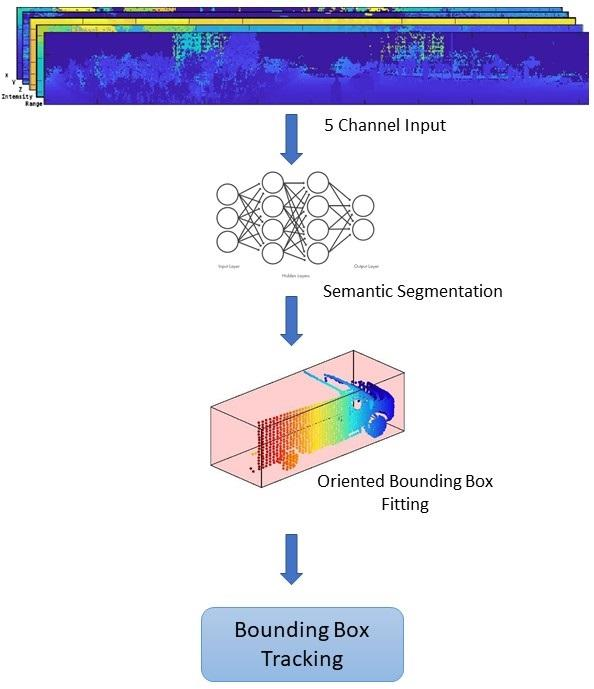The lidar sensor generates point cloud data either in an organized format or an unorganized format. The data used in this example is collected using an Ouster OS1 lidar sensor. This lidar produces an organized point cloud with 64 horizontal scan lines. The point cloud data is comprised of three channels, representing the x-, y-, and z-coordinates of the points. Each channel is of the size 64-by-1024. Use the helper function `helperDownloadData` to download the data and load them into the MATLAB® workspace.

`[ptClouds,pretrainedModel] = helperDownloadData;`

### Ground Plane Segmentation

This example employs a hybrid approach that uses the `segmentGroundFromLidarData` and `pcfitplane` functions. First, estimate the ground plane parameters using the `segmentGroundFromLidarData` function. The estimated ground plane is divided into strips along the direction of the vehicle in order to fit the plane, using the `pcfitplane` function on each strip. This hybrid approach robustly fits the ground plane in a piecewise manner and handles variations in the point cloud.

```% Load point cloud ptCloud = ptClouds{1}; % Define ROI for cropping point cloud xLimit = [-30,30]; yLimit = [-12,12]; zLimit = [-3,15]; roi = [xLimit,yLimit,zLimit]; % Extract ground plane [nonGround,ground] = helperExtractGround(ptCloud,roi); figure; pcshowpair(nonGround,ground); legend({'\color{white} Nonground','\color{white} Ground'},'Location','northeastoutside');```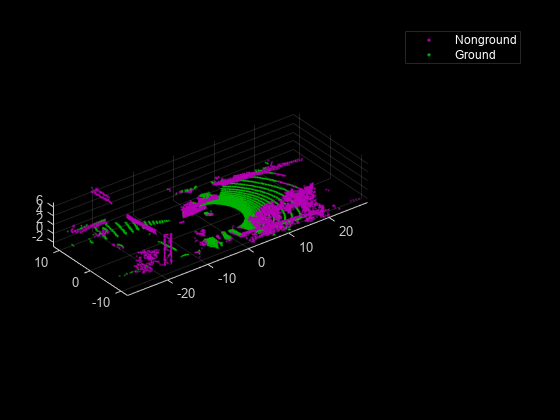### Semantic Segmentation

This example uses a pretrained `PointSeg` network model. `PointSeg` is an end-to-end real-time semantic segmentation network trained for object classes like cars, trucks, and background. The output from the network is a masked image with each pixel labeled per its class. This mask is used to filter different types of objects in the point cloud. The input to the network is five-channel image, that is x, y, z, intensity, and range. For more information on the network or how to train the network, refer to the Lidar Point Cloud Semantic Segmentation Using PointSeg Deep Learning Network example.

#### Prepare Input Data

The `helperPrepareData` function generates five-channel data from the loaded point cloud data.

```% Load and visualize a sample frame frame = helperPrepareData(ptCloud); figure; subplot(5,1,1); imagesc(frame(:,:,1)); title('X channel'); subplot(5,1,2); imagesc(frame(:,:,2)); title('Y channel'); subplot(5,1,3); imagesc(frame(:,:,3)); title('Z channel'); subplot(5,1,4); imagesc(frame(:,:,4)); title('Intensity channel'); subplot(5,1,5); imagesc(frame(:,:,5)); title('Range channel');```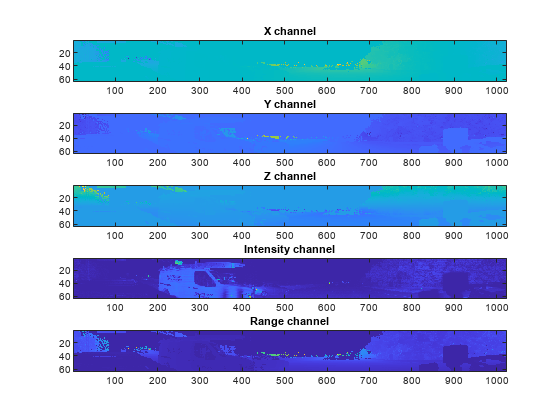Run forward inference on one frame from the loaded pre-trained network.

```if ~exist('net','var') net = pretrainedModel.net; end % Define classes classes = ["background","car","truck"]; % Define color map lidarColorMap = [ 0.98 0.98 0.00 % unknown 0.01 0.98 0.01 % green color for car 0.01 0.01 0.98 % blue color for motorcycle ]; % Run forward pass pxdsResults = semanticseg(frame,net); % Overlay intensity image with segmented output segmentedImage = labeloverlay(uint8(frame(:,:,4)),pxdsResults,'Colormap',lidarColorMap,'Transparency',0.5); % Display results figure; imshow(segmentedImage); helperPixelLabelColorbar(lidarColorMap,classes);```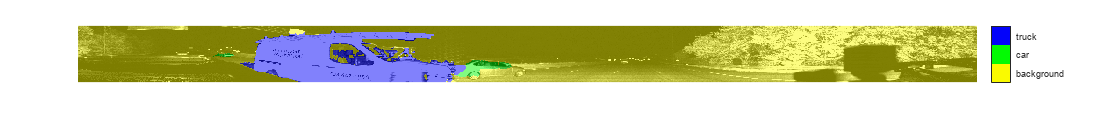Use the generated semantic mask to filter point clouds containing trucks. Similarly, filter point clouds for other classes.

```truckIndices = pxdsResults == 'truck'; truckPointCloud = select(nonGround,truckIndices,'OutputSize','full'); % Crop point cloud for better display croppedPtCloud = select(ptCloud,findPointsInROI(ptCloud,roi)); croppedTruckPtCloud = select(truckPointCloud,findPointsInROI(truckPointCloud,roi)); % Display ground and nonground points figure; pcshowpair(croppedPtCloud,croppedTruckPtCloud); legend({'\color{white} Nonvehicle','\color{white} Vehicle'},'Location','northeastoutside');```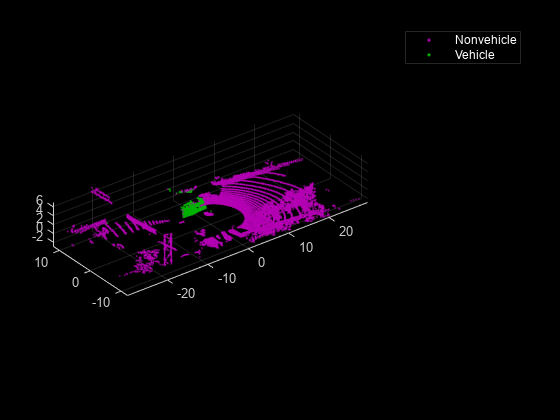### Clustering and Bounding Box Fitting

After extracting point clouds of different object classes, the objects are clustered by applying Euclidean clustering using the `pcsegdist` function. To group all the points belonging to one single cluster, the point cloud obtained as a cluster is used as seed points for growing region in nonground points. Use the `findNearestNeighbors` function to loop over all the points to grow the region. The extracted cluster is fitted in an L-shape bounding box using the `pcfitcuboid` function. These clusters of vehicles resemble the shape of the letter L when seen from a top-down view. This feature helps in estimating the orientation of the vehicle. The oriented bounding box fitting helps in estimating the heading angle of the objects, which is useful in applications such as path planning and traffic maneuvering.

The cuboid boundaries of the clusters can also be calculated by finding the minimum and maximum spatial extents in each direction. However, this method fails in estimating the orientation of the detected vehicles. The difference between the two methods is shown in the figure.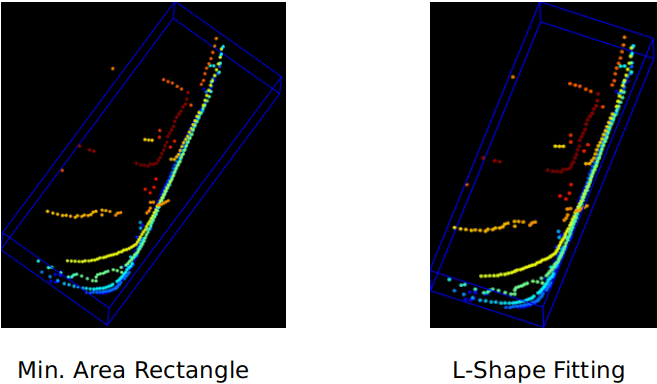```[labels,numClusters] = pcsegdist(croppedTruckPtCloud,1); % Define cuboid parameters params = zeros(0,9); for clusterIndex = 1:numClusters ptsInCluster = labels == clusterIndex; pc = select(croppedTruckPtCloud,ptsInCluster); location = pc.Location; xl = (max(location(:,1)) - min(location(:,1))); yl = (max(location(:,2)) - min(location(:,2))); zl = (max(location(:,3)) - min(location(:,3))); % Filter small bounding boxes if size(location,1)*size(location,2) > 20 && any(any(pc.Location)) && xl > 1 && yl > 1 indices = zeros(0,1); objectPtCloud = pointCloud(location); for i = 1:size(location,1) seedPoint = location(i,:); indices(end+1) = findNearestNeighbors(nonGround,seedPoint,1); end % Remove overlapping indices indices = unique(indices); % Fit oriented bounding box model = pcfitcuboid(select(nonGround,indices)); params(end+1,:) = model.Parameters; end end % Display point cloud and detected bounding box figure; pcshow(croppedPtCloud.Location,croppedPtCloud.Location(:,3)); showShape('cuboid',params,"Color","red","Label","Truck");```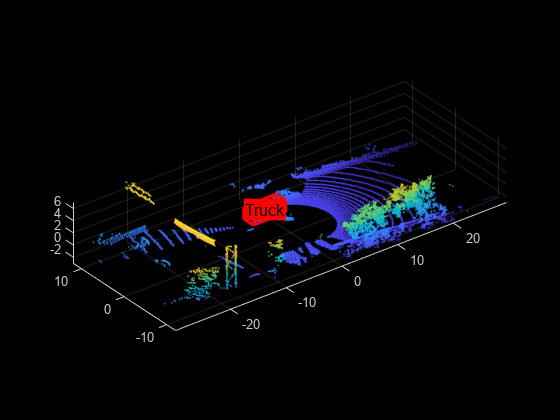### Visualization Setup

Use the `helperLidarObjectDetectionDisplay` class to visualize the complete workflow in one window. The layout of the visualization window is divided into the following sections:

1. Lidar Range Image: point cloud image in 2-D as a range image

2. Segmented Image: Detected labels generated from the semantic segmentation network overlaid with the intensity image or the fourth channel of the data

3. Oriented Bounding Box Detection: 3-D point cloud with oriented bounding boxes

4. Top View: Top view of the point cloud with oriented bounding boxes

`display = helperLidarObjectDetectionDisplay;`

### Loop Through Data

The `helperLidarObjectDetection` class is a wrapper encapsulating all the segmentation, clustering, and bounding box fitting steps mentioned in the above sections. Use the `findDetections` function to extract the detected objects.

```% Initialize lidar object detector lidarDetector = helperLidarObjecDetector('Model',net,'XLimits',xLimit,... 'YLimit',yLimit,'ZLimit',zLimit); % Prepare 5-D lidar data inputData = helperPrepareData(ptClouds); % Set random number generator for reproducible results S = rng(2018); % Initialize the display initializeDisplay(display); numFrames = numel(inputData); for count = 1:numFrames % Get current data input = inputData{count}; rangeImage = input(:,:,5); % Extact bounding boxes from lidar data [boundingBox,coloredPtCloud,pointLabels] = detectBbox(lidarDetector,input); % Update display with colored point cloud updatePointCloud(display,coloredPtCloud); % Update bounding boxes updateBoundingBox(display,boundingBox); % Update segmented image updateSegmentedImage(display,pointLabels,rangeImage); drawnow('limitrate'); end```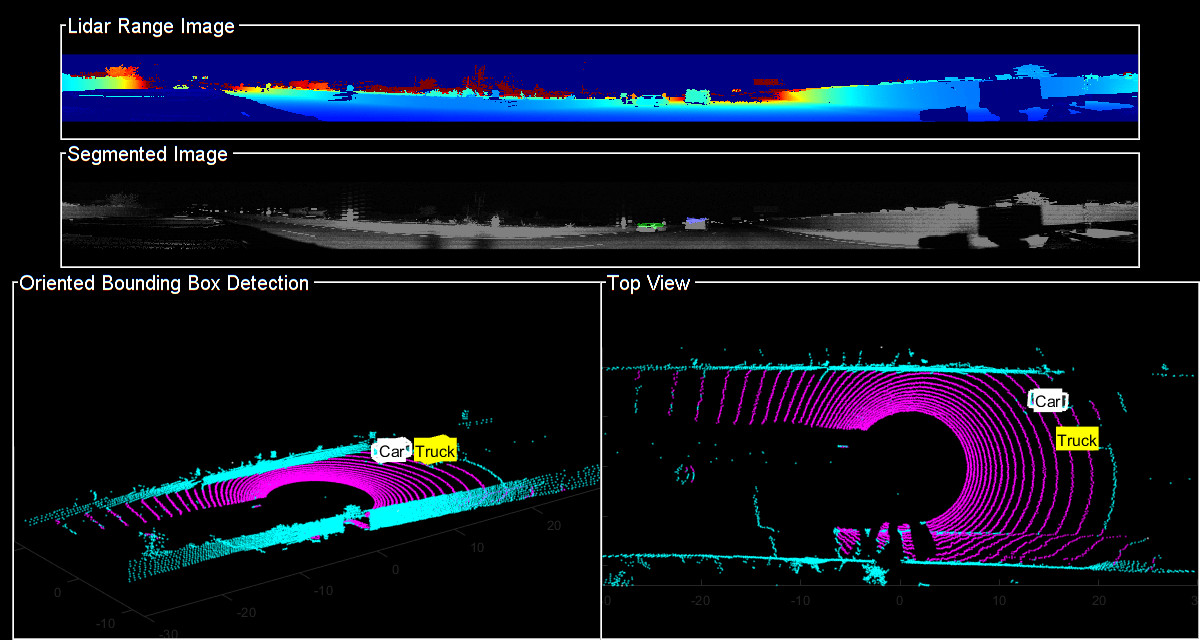### Tracking Oriented Bounding Boxes

In this example, you use a joint probabilistic data association (JPDA) tracker. The time step `dt` is set to 0.1 seconds since the dataset is captured at 10 Hz. The state-space model used in the tracker is based on a cuboid model with parameters, $\left[\mathit{x},\text{\hspace{0.17em}}\mathit{y},\text{\hspace{0.17em}}\mathit{z},\text{\hspace{0.17em}}\varphi ,\text{\hspace{0.17em}}\mathit{l},\text{\hspace{0.17em}}\mathit{w},\text{\hspace{0.17em}}\mathit{h}\right]$. For more details on how to track bounding boxes in lidar data, see the Track Vehicles Using Lidar: From Point Cloud to Track List (Sensor Fusion and Tracking Toolbox) example. In this example, the class information is provided using the `ObjectAttributes `property of the `objectDetection object`. When creating new tracks, the filter initialization function, defined using the helper function `helperMultiClassInitIMMFilter` uses the class of the detection to set up initial dimensions of the object. This helps the tracker to adjust bounding box measurement model with the appropriate dimensions of the track.

Set up a JPDA tracker object with these parameters.

```assignmentGate = [10 100]; % Assignment threshold; confThreshold = [7 10]; % Confirmation threshold for history logi delThreshold = [2 3]; % Deletion threshold for history logic Kc = 1e-5; % False-alarm rate per unit volume % IMM filter initialization function filterInitFcn = @helperMultiClassInitIMMFilter; % A joint probabilistic data association tracker with IMM filter tracker = trackerJPDA('FilterInitializationFcn',filterInitFcn,... 'TrackLogic','History',... 'AssignmentThreshold',assignmentGate,... 'ClutterDensity',Kc,... 'ConfirmationThreshold',confThreshold,... 'DeletionThreshold',delThreshold,'InitializationThreshold',0); allTracks = struct([]); time = 0; dt = 0.1; % Define Measurement Noise measNoise = blkdiag(0.25*eye(3),25,eye(3)); numTracks = zeros(numFrames,2);```

The detected objects are assembled as a cell array of `objectDetection` (Automated Driving Toolbox) objects using the `helperAssembleDetections` function.

```display = helperLidarObjectDetectionDisplay; initializeDisplay(display); for count = 1:numFrames time = time + dt; % Get current data input = inputData{count}; rangeImage = input(:,:,5); % Extact bounding boxes from lidar data [boundingBox,coloredPtCloud,pointLabels] = detectBbox(lidarDetector,input); % Assemble bounding boxes into objectDetections detections = helperAssembleDetections(boundingBox,measNoise,time); % Pass detections to tracker if ~isempty(detections) % Update the tracker [confirmedTracks,tentativeTracks,allTracks,info] = tracker(detections,time); numTracks(count,1) = numel(confirmedTracks); end % Update display with colored point cloud updatePointCloud(display,coloredPtCloud); % Update segmented image updateSegmentedImage(display,pointLabels,rangeImage); % Update the display if the tracks are not empty if ~isempty(confirmedTracks) updateTracks(display,confirmedTracks); end drawnow('limitrate'); end```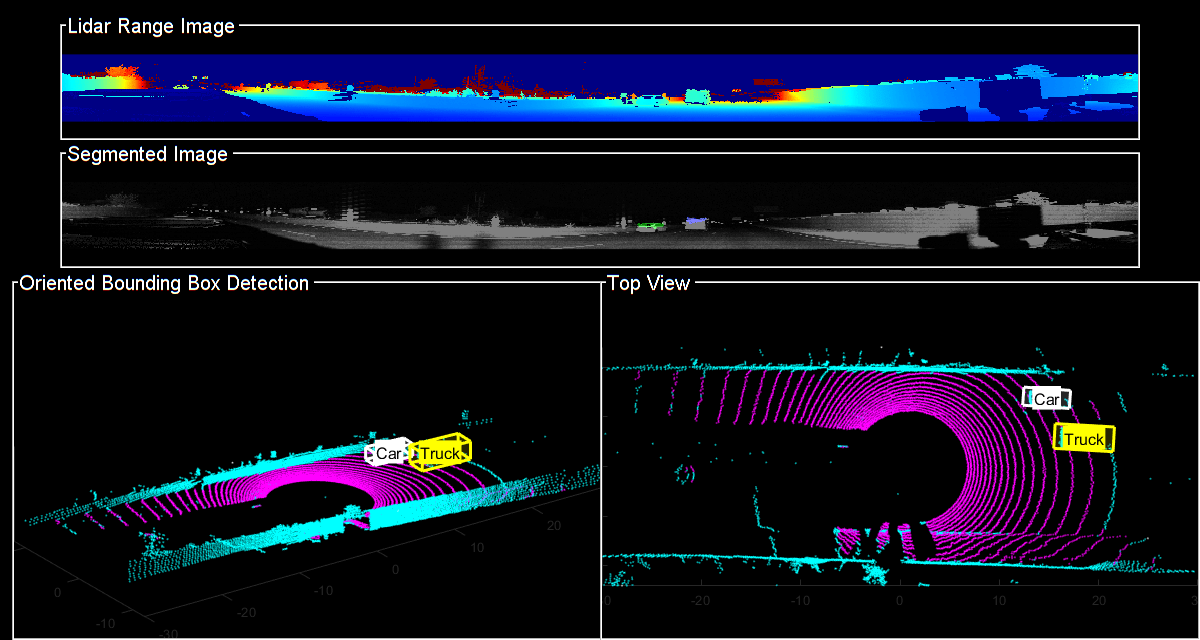### Summary

This example showed how to detect and classify vehicles fitted with oriented bounding box on lidar data. You also learned how to use IMM filter to track objects with multiple class information. The semantic segmentation results can be improved further by adding more training data.

### Supporting Functions

#### helperPrepareData

```function multiChannelData = helperPrepareData(input) % Create 5-channel data as x, y, z, intensity and range % of size 64-by-1024-by-5 from pointCloud. if isa(input, 'cell') numFrames = numel(input); multiChannelData = cell(1, numFrames); for i = 1:numFrames inputData = input{i}; x = inputData.Location(:,:,1); y = inputData.Location(:,:,2); z = inputData.Location(:,:,3); intensity = inputData.Intensity; range = sqrt(x.^2 + y.^2 + z.^2); multiChannelData{i} = cat(3, x, y, z, intensity, range); end else x = input.Location(:,:,1); y = input.Location(:,:,2); z = input.Location(:,:,3); intensity = input.Intensity; range = sqrt(x.^2 + y.^2 + z.^2); multiChannelData = cat(3, x, y, z, intensity, range); end end```

#### pixelLabelColorbar

```function helperPixelLabelColorbar(cmap, classNames) % Add a colorbar to the current axis. The colorbar is formatted % to display the class names with the color. colormap(gca,cmap) % Add colorbar to current figure. c = colorbar('peer', gca); % Use class names for tick marks. c.TickLabels = classNames; numClasses = size(cmap,1); % Center tick labels. c.Ticks = 1/(numClasses*2):1/numClasses:1; % Remove tick mark. c.TickLength = 0; end```

#### helperExtractGround

```function [ptCloudNonGround,ptCloudGround] = helperExtractGround(ptCloudIn,roi) % Crop the point cloud idx = findPointsInROI(ptCloudIn,roi); pc = select(ptCloudIn,idx,'OutputSize','full'); % Get the ground plane the indices using piecewise plane fitting [ptCloudGround,idx] = piecewisePlaneFitting(pc,roi); nonGroundIdx = true(size(pc.Location,[1,2])); nonGroundIdx(idx) = false; ptCloudNonGround = select(pc,nonGroundIdx,'OutputSize','full'); end function [groundPlane,idx] = piecewisePlaneFitting(ptCloudIn,roi) groundPtsIdx = ... segmentGroundFromLidarData(ptCloudIn, ... 'ElevationAngleDelta',5,'InitialElevationAngle',15); groundPC = select(ptCloudIn,groundPtsIdx,'OutputSize','full'); % Divide x-axis in 3 regions segmentLength = (roi(2) - roi(1))/3; x1 = [roi(1),roi(1) + segmentLength]; x2 = [x1(2),x1(2) + segmentLength]; x3 = [x2(2),x2(2) + segmentLength]; roi1 = [x1,roi(3:end)]; roi2 = [x2,roi(3:end)]; roi3 = [x3,roi(3:end)]; idxBack = findPointsInROI(groundPC,roi1); idxCenter = findPointsInROI(groundPC,roi2); idxForward = findPointsInROI(groundPC,roi3); % Break the point clouds in front and back ptBack = select(groundPC,idxBack,'OutputSize','full'); ptForward = select(groundPC,idxForward,'OutputSize','full'); [~,inliersForward] = planeFit(ptForward); [~,inliersBack] = planeFit(ptBack); idx = [inliersForward; idxCenter; inliersBack]; groundPlane = select(ptCloudIn, idx,'OutputSize','full'); end function [plane,inlinersIdx] = planeFit(ptCloudIn) [~,inlinersIdx, ~] = pcfitplane(ptCloudIn,1,[0, 0, 1]); plane = select(ptCloudIn,inlinersIdx,'OutputSize','full'); end```

#### helperAssembleDetections

```function mydetections = helperAssembleDetections(bboxes,measNoise,timestamp) % Assemble bounding boxes as cell array of objectDetection mydetections = cell(size(bboxes,1),1); for i = 1:size(bboxes,1) classid = bboxes(i,end); lidarModel = [bboxes(i,1:3), bboxes(i,end-1), bboxes(i,4:6)]; % To avoid direct confirmation by the tracker, the ClassID is passed as % ObjectAttributes. mydetections{i} = objectDetection(timestamp, ... lidarModel','MeasurementNoise',... measNoise,'ObjectAttributes',struct('ClassID',classid)); end end```

```function [lidarData, pretrainedModel] = helperDownloadData outputFolder = fullfile(tempdir,'WPI'); url = 'https://ssd.mathworks.com/supportfiles/lidar/data/lidarSegmentationAndTrackingData.tar.gz'; lidarDataTarFile = fullfile(outputFolder,'lidarSegmentationAndTrackingData.tar.gz'); if ~exist(lidarDataTarFile,'file') mkdir(outputFolder); websave(lidarDataTarFile,url); untar(lidarDataTarFile,outputFolder); end % Check if tar.gz file is downloaded, but not uncompressed if ~exist(fullfile(outputFolder,'WPI_LidarData.mat'),'file') untar(lidarDataTarFile,outputFolder); end % Load lidar data data = load(fullfile(outputFolder,'highwayData.mat')); lidarData = data.ptCloudData; % Download pretrained model url = 'https://ssd.mathworks.com/supportfiles/lidar/data/pretrainedPointSegModel.mat'; modelFile = fullfile(outputFolder,'pretrainedPointSegModel.mat'); if ~exist(modelFile,'file') websave(modelFile,url); end pretrainedModel = load(fullfile(outputFolder,'pretrainedPointSegModel.mat')); end```